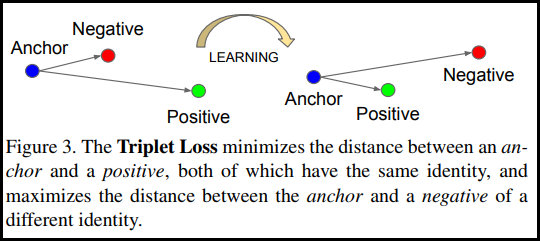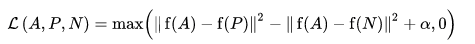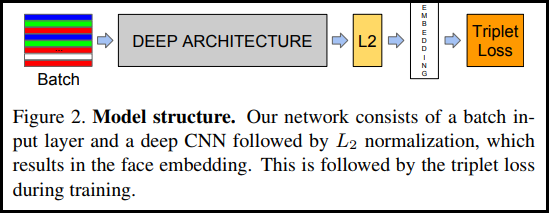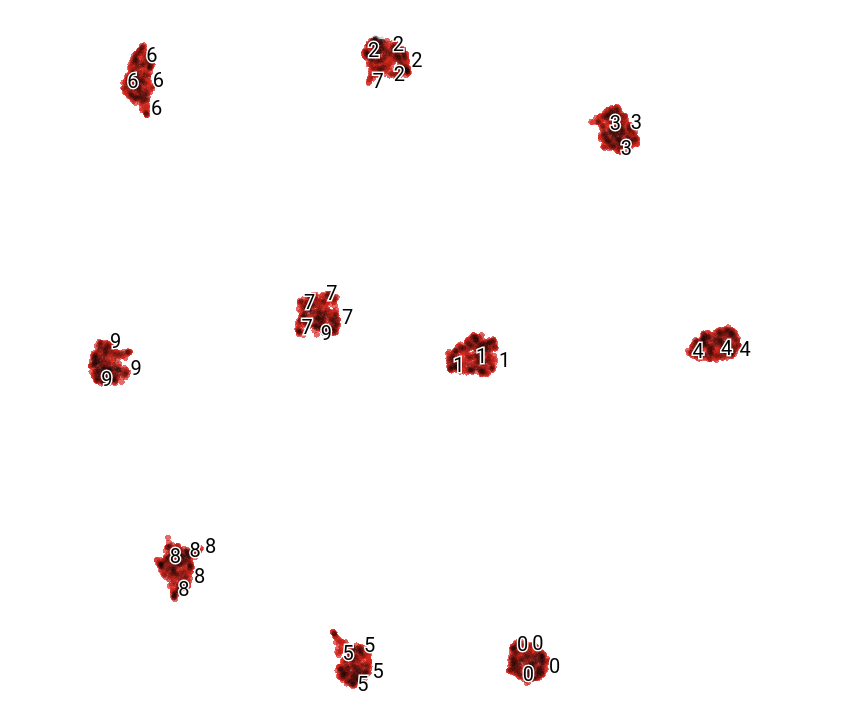## Overview

This notebook will demonstrate how to use the TripletSemiHardLoss function in TensorFlow Addons.

## TripletLoss

As first introduced in the FaceNet paper, TripletLoss is a loss function that trains a neural network to closely embed features of the same class while maximizing the distance between embeddings of different classes. To do this an anchor is chosen along with one negative and one positive sample.The loss function is described as a Euclidean distance function:Where A is our anchor input, P is the positive sample input, N is the negative sample input, and alpha is some margin you use to specify when a triplet has become too "easy" and you no longer want to adjust the weights from it.

## SemiHard Online Learning

As shown in the paper, the best results are from triplets known as "Semi-Hard". These are defined as triplets where the negative is farther from the anchor than the positive, but still produces a positive loss. To efficiently find these triplets you utilize online learning and only train from the Semi-Hard examples in each batch.

## Setup

````pip install -q -U tensorflow-addons`
```
``````import io
import numpy as np
``````
``````import tensorflow as tf
import tensorflow_datasets as tfds
``````

## Prepare the Data

``````def _normalize_img(img, label):
img = tf.cast(img, tf.float32) / 255.
return (img, label)

train_dataset, test_dataset = tfds.load(name="mnist", split=['train', 'test'], as_supervised=True)

train_dataset = train_dataset.shuffle(1024).batch(32)
train_dataset = train_dataset.map(_normalize_img)

test_dataset = test_dataset.batch(32)
test_dataset = test_dataset.map(_normalize_img)
``````
```Downloading and preparing dataset 11.06 MiB (download: 11.06 MiB, generated: 21.00 MiB, total: 32.06 MiB) to /home/kbuilder/tensorflow_datasets/mnist/3.0.1...
Dataset mnist downloaded and prepared to /home/kbuilder/tensorflow_datasets/mnist/3.0.1. Subsequent calls will reuse this data.
```

## Build the Model``````model = tf.keras.Sequential([
tf.keras.layers.MaxPooling2D(pool_size=2),
tf.keras.layers.Dropout(0.3),
tf.keras.layers.MaxPooling2D(pool_size=2),
tf.keras.layers.Dropout(0.3),
tf.keras.layers.Flatten(),
tf.keras.layers.Dense(256, activation=None), # No activation on final dense layer
tf.keras.layers.Lambda(lambda x: tf.math.l2_normalize(x, axis=1)) # L2 normalize embeddings

])
``````

## Train and Evaluate

``````# Compile the model
model.compile(
loss=tfa.losses.TripletSemiHardLoss())
``````
``````# Train the network
history = model.fit(
train_dataset,
epochs=5)
``````
```Epoch 1/5
1875/1875 [==============================] - 21s 5ms/step - loss: 0.6983
Epoch 2/5
1875/1875 [==============================] - 8s 4ms/step - loss: 0.4723
Epoch 3/5
1875/1875 [==============================] - 8s 4ms/step - loss: 0.4298
Epoch 4/5
1875/1875 [==============================] - 8s 4ms/step - loss: 0.4139
Epoch 5/5
1875/1875 [==============================] - 8s 4ms/step - loss: 0.3938
```
``````# Evaluate the network
results = model.predict(test_dataset)
``````
``````# Save test embeddings for visualization in projector
np.savetxt("vecs.tsv", results, delimiter='\t')

out_m = io.open('meta.tsv', 'w', encoding='utf-8')
for img, labels in tfds.as_numpy(test_dataset):
[out_m.write(str(x) + "\n") for x in labels]
out_m.close()

try:
You can see the results of our embedded test data when visualized with UMAP: